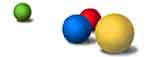This morning there was an intriguing post on arXiv/math.RA
entitled A Note on
the Eigenvalues of the Google Matrix
. At first I thought it was a
joke but a quick Google revealed that the PageRank algorithm really
is at the heart of Google technology, so I simply had to find out more
about it. An extremely readable account of it can be found in The PageRank Citation Ranking: Bringing Order to the Web which is really the
start of Google. It is coauthored by the two founders : Larry Page and
Sergey Brin. A quote from the introduction

“To test the utility of PageRank for search, we built a web
5)”

Here is an intuitive idea of
_PageRank_ : a page has high rank if the sum of the ranks of its
high and it is computed by the _Random Surfer Model_ (see
sections 2.5 and 2.6 of the paper). More formally (at least from my
quick browsing of some papers, maybe the following account is slightly
erroneous and I’ll have to spend some more time reading) let
N be the number of webpages (estimated between 3 and 4
billion) and consider the N x N matrix
A the so called GoogleMatrix where

A = cP  + (1-c)(v x
vec(1)) 

where P is the
column-stochastic matrix (meaning : all entries are zero or positive and
the sum of all entries in each column adds up to 1) with
entries

P(i,j) = 1/N(i) if i->j and 0
otherwise 

where i and j are webpages and i->j
denotes that page i has a link to page j and where N(i) is the total
number of pages linked to in page i (all this information is available
once we download page i). c is a constant 0 < c < 1 and
corresponds to the fraction of webpages containing an _outlink (that
is, a link to another page) by all webpages (it seems that Google uses
c=0.85 as an estimate). Finally, v is a column vector with zero or
positive numbers adding up to 1 and vec(1) is the constant row vector
(1,…,1). The idea behind this term is that in the _Random Surfer
Model_ to compute the PageRank the Googlebot (normally following
to an entirely different webpage where the chance that it will end up at
page i is given by the i-th entry of v (this is to avoid being trapped
in a web-loop). So, in Googles model the bot _teleports_ itself
randomly every 6th link or so. Now, the PageRank is a
column-eigenvector for the GoogleMatrix A with eigenvalue 1 which can be
approximated by the RandomSurfer model and the rate of convergence of
this process depends on the _second_ largest eigenvalue for A
(the largest being 1). Now, in the paper posted this morning a simple
proof is given that this eigenvalue is c (because the matrix P has
multiple eigenvalues equal to 1). According to a previous paper on the
subject The
Second Eigenvalue of the Google Matrix
, this statement has
implications for the convergence rate of the standard PageRank algorithm
as the web scales, for the stability of PageRank to perturbations to the
link structure of the web, for the detection of Google spammers, and for
the design of algorithms to speed up PageRank. But I’ll have to